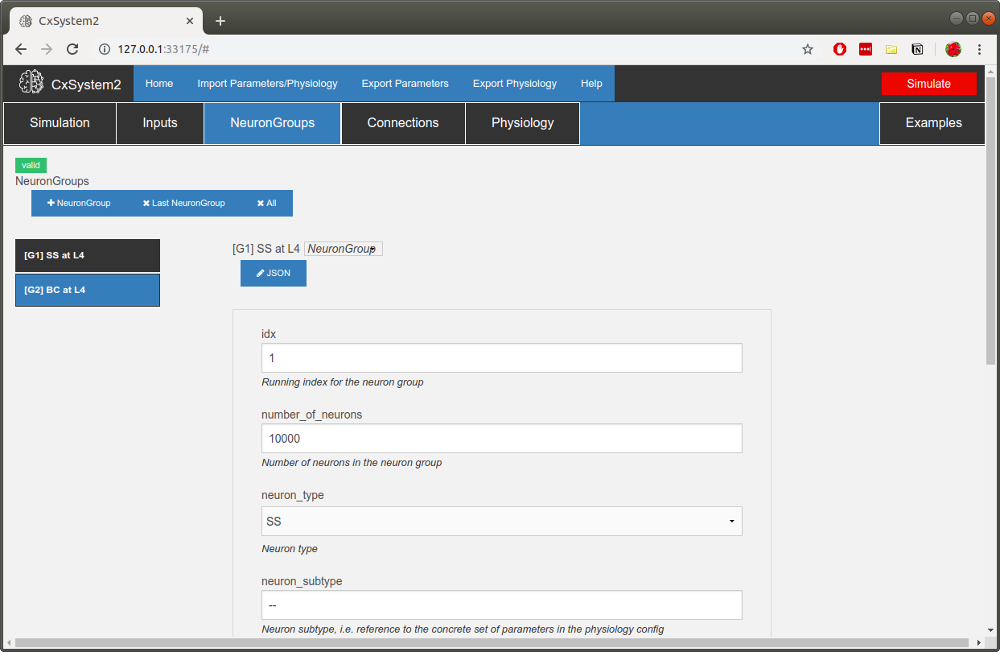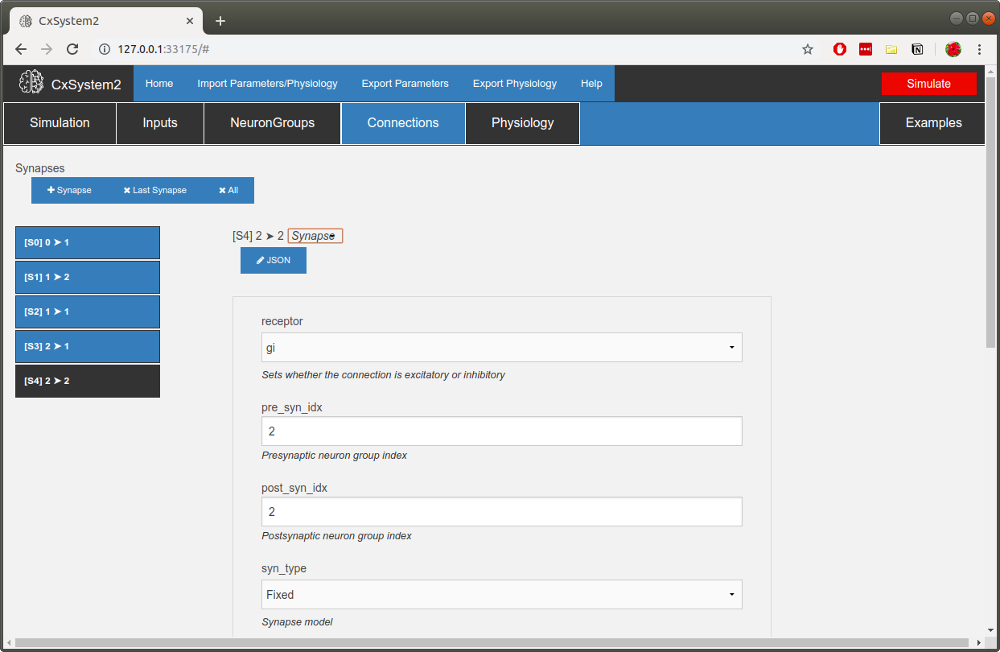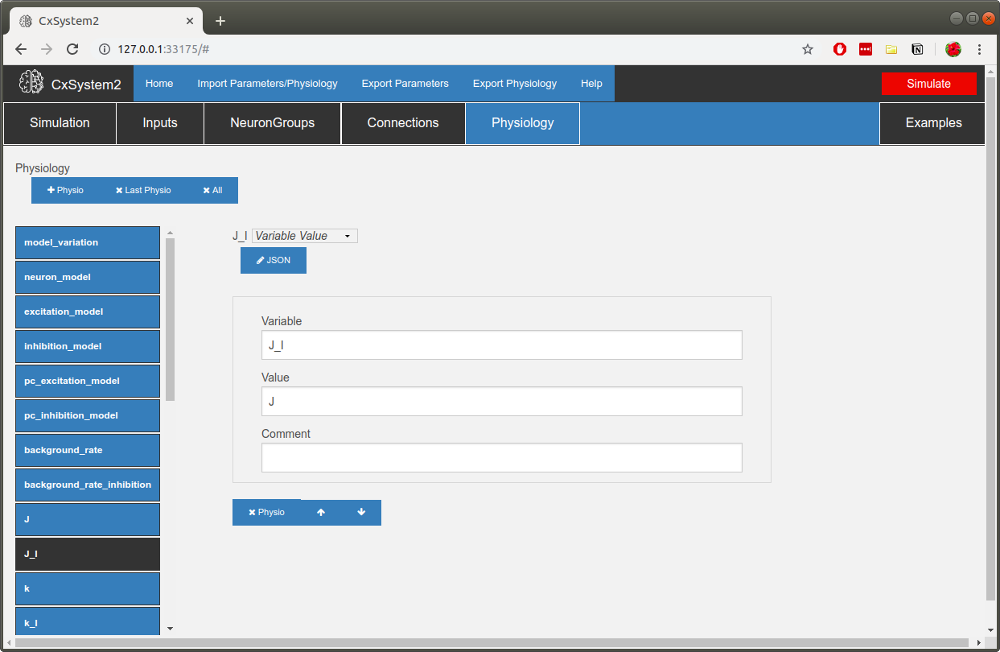# 3 - Building a new model¶

Let’s then build a new network composed of adaptive exponential integrate-and-fire neurons. First, open the Template network under the Examples tab.

Let’s first set the general simulation parameters. You don’t need to worry about many of the things here for now. Just change the `simulation_title` from the template title. Also, check that `init_vms` (we want to randomize initial membrane voltages) and `multidimension_array_run` are checked (we want to do a parameter search). Run_in_cluster should be left unchecked. Finally set the `runtime` parameter (topmost) to 3*second and `device` to Cython.

Now, click the NeuronGroups tab. In the template config, there is one excitatory and one inhibitory population, and this is the same setup we will be using here. Let’s however increase the neuron counts a bit. Pick the spiny stellate (SS) group and change the number of neurons to 10000:Also change the number of background inputs to 1000. For the basket cell (BC) group, change the number of neurons to 2500 and the number of background inputs to 1000.

Now, let’s add some connections. We need to remember here that neuron index 1 corresponds to the excitatory group and neuron index 2 correspond to the inhibitory group. Let’s leave the first connection `S0` as it is (this is a connection from the input group). Now open up `S1` and from there change the number of synapses per connection (parameter `n`) to 1. Let’s leave the connection probability (parameter `p`) at 10% (0.1).

Let’s add the rest of the connections. Click the +Synapse button. Then set the parameters for `S2`: we want this to be an excitatory connection (set `receptor` to `ge`) from the excitatory group to itself (set `pre_syn_idx` to 1 and `post_syn_idx` to 1). Set `p` to 0.1 and `n` to 1 again. The rest of the parameters can be left as they are by default.

Now add the inhibitory connections. Click the +Synapse button again. Set the parameters for `S3`: we want this to be an inhibitory connection (set `receptor` to `gi`) from the inhibitory group to the excitatory group (set `pre_syn_idx` to 2 and `post_syn_idx` to 1). Set `p` to 0.1 and `n` to 1 again. Finally, add an inhibitory self-connection with the same `p` and `n`. You should have a collection of synapses like this:We’re almost there… but we still need to set parameters under the Physiology tab. Because we want to simulate AdEx neurons you need to change the `neuron_model` parameter to `'ADEX'`. (Note that you need to have single- or double-quotes around the model name – otherwise CxSystem will think you are referring to a constant in the configuration file.) Check that `excitation_model` is `'SIMPLE_E'` and `inhibition_model` is `'SIMPLE_I'` (exponentially decaying conductance for excitatory and inhibitory receptors). There are some parameters that are not used in this simulation (like `pc_excitation_model` which is for pyramidal cells), but you don’t need to delete these parameters.

A common scheme to set connection weights is to set weights to excitatory connections and then specify the inhibitory connections with respect to them. Thus, in this template, there are global variables for each of these: `J` (E-to-E connections), `J_I` (E-to-I connections), `k` (ratio of I-E connection weight with respect to E-to-E connection weight) and `k_I` (ratio of I-to-I connection weight with respect to E-to-I connection weight). We will only be needing one excitatory weight and one inhibitory weight. Set `J` to 0.5*nS. Then set `J_I` to J and `k_I` to k:Can you find where the time constants for the receptors are? They are under `receptor_taus` (the letter tau often signifies a time constant). Change `tau_e` to 5*ms and `tau_i` to 10*ms.

Now we still need to change the neuron model parameters. Go to the bottom of the list of parameters and open up the BC dictionary. Inside you’ll find parameters for the BC neuron, which in the template are EIF neurons. We need to add some parameters to parametrize AdEx neurons. First, however, change the existing parameters: `C` to 59*pF, `gL` to 2.9*nS, `EL` to -62*mV, `VT` to -42*mV, `DeltaT` to 3*mV and `V_res` to -54*mV. Then scroll down to the bottom and click the +row button three times. Then in the new empty fields write down the new parameter values: `a` = 1.8*nS, `b` = 61*pA and `tau_w` = 16*ms. Finally, change the `V_init_min` parameter to EL-5*mV and `V_init_max` to EL+5*mV.

Once you have done all the changes to the BC neuron group, do the same changes to the SS group.

Now we should have a working simulation config. But what’s the parameter search we wanted to do? We want to see how changing background input rate and changing the ratio of inhibition-to-excitation changes changes network behavior. There are two notations for parameter search in CxSystem. The first notation is `{start|end|step}` which has a similar behavior as `numpy.arange(start,end,step)`. For example, setting a parameter to `{0|1|0.2}` would create an array of following values: `0.0, 0.2, 0.4, 0.6, 0.8`. The next notation is `{value1 & value2 & value3 & ... }` where the user can add multiple desired values manually. For example, setting a parameter to `{ 0 & 1 & 100 & 9 }` will create an array of following values: `0, 1 , 100, 9`. Note that these values can be used at the same time. As an example, consider we want to add search ranges to the parameters `background_rate` and `k`. Here we use the curly brace syntax: set `background_rate` as {0|5|2}*Hz (corresponding to 0, 2, 4 Hz) and `k` as {1 & 3 & 5 & 7}. Now you have defined a 3x4 parameter search of following values:

 background_rate 0 0 0 0 2 2 2 2 4 4 4 4 k 1 3 5 7 1 3 5 7 1 3 5 7

Finally you can hit the Simulate button. When you simulate this on a single CPU core (`number_of_process` is 1) it should take 30 min - 1 hour to finish. Once the simulation is finished you should be able to see 12 files in your workspace:

```my_first_model_results_20191216_0901136_background_rate0H_k1.gz
my_first_model_results_20191216_0901136_background_rate0H_k3.gz
my_first_model_results_20191216_0901136_background_rate0H_k5.gz
my_first_model_results_20191216_0901136_background_rate0H_k7.gz
my_first_model_results_20191216_0901136_background_rate2H_k1.gz
my_first_model_results_20191216_0901136_background_rate2H_k3.gz
my_first_model_results_20191216_0901136_background_rate2H_k5.gz
my_first_model_results_20191216_0901136_background_rate2H_k7.gz
my_first_model_results_20191216_0901136_background_rate4H_k1.gz
my_first_model_results_20191216_0901136_background_rate4H_k3.gz
my_first_model_results_20191216_0901136_background_rate4H_k5.gz
my_first_model_results_20191216_0901136_background_rate4H_k7.gz
```

Now you can use the techniques described in Tutorial 3 to visualize the results!